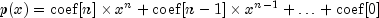JMSLTM Numerical Library 7.2.0
com.imsl.math

## Class ZeroPolynomial

• All Implemented Interfaces:
Serializable, Cloneable

```public class ZeroPolynomial
extends Object
implements Serializable, Cloneable```
The ZeroPolynomial class computes the zeros of a polynomial with complex coefficients, Aberth's method. This class is a Java translation of a Fortran code written by Dario Andrea Bini, University of Pisa, Italy (bini@dm.unipi.it). Numerical computation of polynomial zeros by means of Aberth's method, Numerical Algorithms, 13 (1996), pp. 179-200. The original Fortran code includes the following notice.

All the software contained in this library is protected by copyright Permission to use, copy, modify, and distribute this software for any purpose without fee is hereby granted, provided that this entire notice is included in all copies of any software which is or includes a copy or modification of this software and in all copies of the supporting documentation for such software.

THIS SOFTWARE IS BEING PROVIDED "AS IS", WITHOUT ANY EXPRESS OR IMPLIED WARRANTY. IN NO EVENT, NEITHER THE AUTHORS, NOR THE PUBLISHER, NOR ANY MEMBER OF THE EDITORIAL BOARD OF THE JOURNAL "NUMERICAL ALGORITHMS", NOR ITS EDITOR-IN-CHIEF, BE LIABLE FOR ANY ERROR IN THE SOFTWARE, ANY MISUSE OF IT OR ANY DAMAGE ARISING OUT OF ITS USE. THE ENTIRE RISK OF USING THE SOFTWARE LIES WITH THE PARTY DOING SO. ANY USE OF THE SOFTWARE CONSTITUTES ACCEPTANCE OF THE TERMS OF THE ABOVE STATEMENT.

Real coefficients Example, Complex coefficients Example, Serialized Form
• ### Nested Class Summary

Nested Classes
Modifier and Type Class and Description
`static class ` `ZeroPolynomial.DidNotConvergeException`
The iteration did not converge
• ### Field Summary

Fields
Modifier and Type Field and Description
`static double` `EPSILON_SMALL`
The smallest relative spacing for doubles.
• ### Constructor Summary

Constructors
Constructor and Description
`ZeroPolynomial()`
Creates an instance of the solver.
• ### Method Summary

Methods
Modifier and Type Method and Description
`Complex[]` `computeRoots(Complex[] coef)`
Computes the roots of the polynomial with Complex coefficients.
`Complex[]` `computeRoots(double[] coef)`
Computes the roots of the polynomial with real coefficients.
`double` `getRadius(int index)`
Returns an a-posteriori absolute error bound on the root.
`Complex` `getRoot(int index)`
Returns a zero of the polynomial.
`Complex[]` `getRoots()`
Returns the zeros of the polynomial.
`boolean` `getStatus(int index)`
Returns the error status of a root.
`void` `setMaxIterations(int maxIterations)`
Sets the maximum number of iterations allowed.
• ### Methods inherited from class java.lang.Object

`clone, equals, finalize, getClass, hashCode, notify, notifyAll, toString, wait, wait, wait`
• ### Field Detail

• #### EPSILON_SMALL

`public static final double EPSILON_SMALL`
The smallest relative spacing for doubles.
Constant Field Values
• ### Constructor Detail

• #### ZeroPolynomial

`public ZeroPolynomial()`
Creates an instance of the solver.
• ### Method Detail

• #### computeRoots

```public Complex[] computeRoots(Complex[] coef)
throws ZeroPolynomial.DidNotConvergeException```
Computes the roots of the polynomial with Complex coefficients.Parameters:
`coef` - a `Complex` array containing the polynomial coefficients.
Returns:
a `Complex` array containing the roots of the polynomial.
Throws:
`ZeroPolynomial.DidNotConvergeException`
• #### computeRoots

```public Complex[] computeRoots(double[] coef)
throws ZeroPolynomial.DidNotConvergeException```
Computes the roots of the polynomial with real coefficients.Parameters:
`coef` - a `double` array containing the polynomial coefficients
Returns:
a `Complex` array containing the roots of the polynomial
Throws:
`ZeroPolynomial.DidNotConvergeException`

`public double getRadius(int index)`
Returns an a-posteriori absolute error bound on the root.
Parameters:
`index` - an `int` specifying the (0-based) index of the root whose error bound is to be returned
Returns:
a `double` representing the error bound on the index-th root. NaN is returned if the corresponding root cannot be represented as floating point due to overflow or underflow or if the roots have not yet been computed.
• #### getRoot

`public Complex getRoot(int index)`
Returns a zero of the polynomial.
Parameters:
`index` - an `int` which specifies the (0-based) index of the root to be returned
Returns:
a `Complex` which represents the index-th root of the polynomial
• #### getRoots

`public Complex[] getRoots()`
Returns the zeros of the polynomial.
Returns:
a `Complex` array containing the roots of the polynomial
• #### getStatus

`public boolean getStatus(int index)`
Returns the error status of a root.
Parameters:
`index` - an `int` representing the (0-based) index of the root whose error status is to be returned
Returns:
a `boolean` representing the error status on the index-th root. It is false if the approximation of the index-th root has been carried out successfully, for example, the computed approximation can be viewed as the exact root of a slightly perturbed polynomial. It is true if more iterations are needed for the index-th root.
• #### setMaxIterations

`public void setMaxIterations(int maxIterations)`
Sets the maximum number of iterations allowed. The default value is 30.
Parameters:
`maxIterations` - an `int` which specifies the maximum number of iterations allowed
Throws:
`IllegalArgumentException` - is thrown if `maxIterations` is less than or equal to zero.
JMSLTM Numerical Library 7.2.0